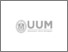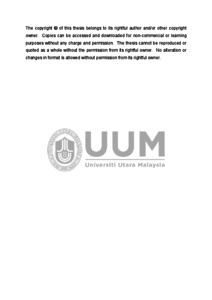UUM Electronic Theses and Dissertation
UUM ETD | Universiti Utara Malaysian Electronic Theses and Dissertation
FAQs | Feedback | Search Tips | Sitemap

# Unrestricted solutions of arbitrary linear fuzzy systems

O.Malkawi, Ghassan (2015) Unrestricted solutions of arbitrary linear fuzzy systems. PhD. thesis, Universiti Utara Malaysia.Text
depositpermission_s93740.pdf
Restricted to Repository staff onlyPreview
Text
s93740_01.pdf

## Abstract

Solving linear fuzzy system has intrigued many researchers due to its ability to handle imprecise information of real problems. However, there are several weaknesses of the existing methods. Among the drawbacks are heavy dependence on linear programing, avoidance of near zero fuzzy numbers, lack of accurate solutions, focus on limited size of the systems, and restriction to the matrix coefficients and solutions. Therefore, this study aims to construct new methods which are associated
linear systems, min-max system and absolute systems in matrix theory with triangular fuzzy numbers to solve linear fuzzy systems with respect to the
aforementioned drawbacks. It is proven that the new constructed associated linear systems are equivalent to linear fuzzy systems without involving any fuzzy operation. Furthermore, the new constructed associated linear systems are effective in providing exact solution as compared to linear programming, which is subjected to a number of constraints. These methods are also able to provide accurate solutions for large systems. Moreover, the existence of fuzzy solutions and classification of
possible solutions are being checked by these associated linear systems. In case of near zero fully fuzzy linear system, fuzzy operations are required to determine the nature of solution of fuzzy system and to ensure the fuzziness of the solution. Finite solutions which are new concept of consistency in linear systems are obtained by the
constructed min-max and absolute systems. These developed methods can also be modified to solve advanced fuzzy systems such as fully fuzzy matrix equation and fully fuzzy Sylvester equation, and can be employed for other types of fuzzy numbers such as trapezoidal fuzzy number. The study contributes to the methods to solve arbitrary linear fuzzy systems without any restriction on the system.

Item Type: Thesis (PhD.) Ahmad, Nazihah and Ibrahim, Haslinda 5779 Arbitrary fully fuzzy linear system, Associated linear system, Matrix theory, Triangular fuzzy number Q Science > QA Mathematics > QA76 Computer software > QA76.76 Fuzzy System. Awang Had Salleh Graduate School of Arts & Sciences 13 Jul 2016 15:02 13 Jul 2016 15:02 Awang Had Salleh Graduate School of Arts and Sciences Ahmad, Nazihah and Ibrahim, Haslinda https://etd.uum.edu.my/id/eprint/5779